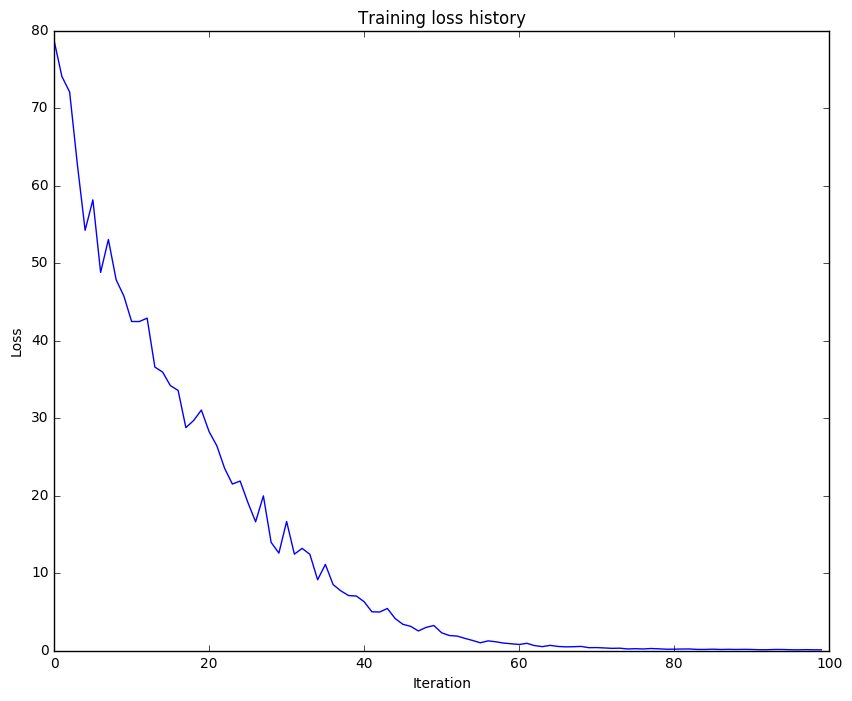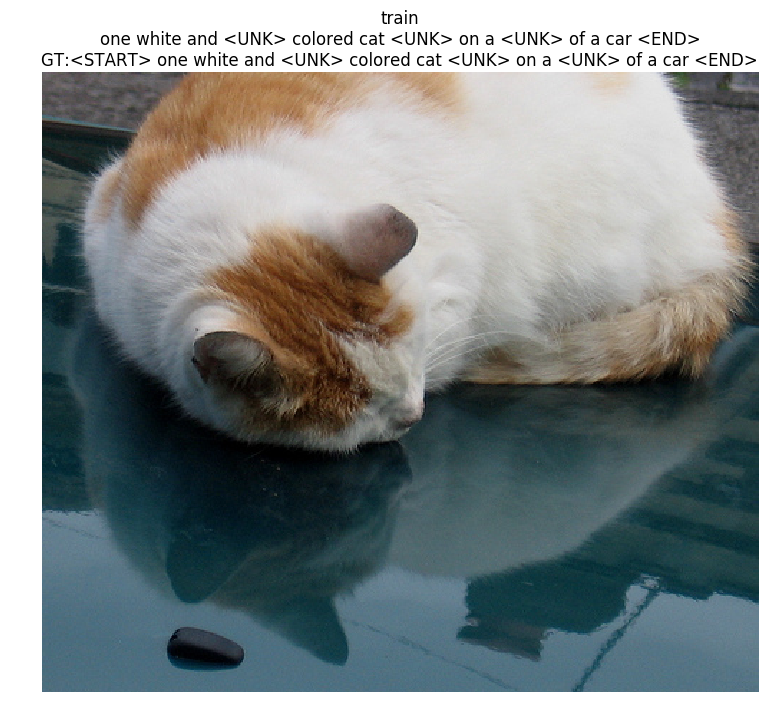LSTM_Captioning

# Image Captioning with LSTMs¶

In this project, we will implement LSTM update rule and use it together with RNN for image captioning.

In :
# As usual, a bit of setup

import time, os, json
import numpy as np
import matplotlib.pyplot as plt

from cs231n.rnn_layers import *
from cs231n.captioning_solver import CaptioningSolver
from cs231n.classifiers.rnn import CaptioningRNN
from cs231n.coco_utils import load_coco_data, sample_coco_minibatch, decode_captions
from cs231n.image_utils import image_from_url

%matplotlib inline
plt.rcParams['figure.figsize'] = (10.0, 8.0) # set default size of plots
plt.rcParams['image.interpolation'] = 'nearest'
plt.rcParams['image.cmap'] = 'gray'

def rel_error(x, y):
""" returns relative error """
return np.max(np.abs(x - y) / (np.maximum(1e-8, np.abs(x) + np.abs(y))))


As in the previous notebook, we will use the Microsoft COCO dataset for captioning.

In :
# Load COCO data from disk; this returns a dictionary
# We'll work with dimensionality-reduced features for this notebook, but feel
# free to experiment with the original features by changing the flag below.

# Print out all the keys and values from the data dictionary
for k, v in data.iteritems():
if type(v) == np.ndarray:
print k, type(v), v.shape, v.dtype
else:
print k, type(v), len(v)

idx_to_word <type 'list'> 1004
train_captions <type 'numpy.ndarray'> (400135, 17) int32
val_captions <type 'numpy.ndarray'> (195954, 17) int32
train_image_idxs <type 'numpy.ndarray'> (400135,) int32
val_features <type 'numpy.ndarray'> (40504, 512) float32
val_image_idxs <type 'numpy.ndarray'> (195954,) int32
train_features <type 'numpy.ndarray'> (82783, 512) float32
train_urls <type 'numpy.ndarray'> (82783,) |S63
val_urls <type 'numpy.ndarray'> (40504,) |S63
word_to_idx <type 'dict'> 1004


# LSTM¶

If you read recent papers, you'll see that many people use a variant on the vanialla RNN called Long-Short Term Memory (LSTM) RNNs. Vanilla RNNs can be tough to train on long sequences due to vanishing and exploding gradiants caused by repeated matrix multiplication. LSTMs solve this problem by replacing the simple update rule of the vanilla RNN with a gating mechanism as follows.

Similar to the vanilla RNN, at each timestep we receive an input $x_t\in\mathbb{R}^D$ and the previous hidden state $h_{t-1}\in\mathbb{R}^H$; the LSTM also maintains an $H$-dimensional cell state, so we also receive the previous cell state $c_{t-1}\in\mathbb{R}^H$. The learnable parameters of the LSTM are an input-to-hidden matrix $W_x\in\mathbb{R}^{4H\times D}$, a hidden-to-hidden matrix $W_h\in\mathbb{R}^{4H\times H}$ and a bias vector $b\in\mathbb{R}^{4H}$.

At each timestep we first compute an activation vector $a\in\mathbb{R}^{4H}$ as $a=W_xx_t + W_hh_{t-1}+b$. We then divide this into four vectors $a_i,a_f,a_o,a_g\in\mathbb{R}^H$ where $a_i$ consists of the first $H$ elements of $a$, $a_f$ is the next $H$ elements of $a$, etc. We then compute the input gate $g\in\mathbb{R}^H$, forget gate $f\in\mathbb{R}^H$, output gate $o\in\mathbb{R}^H$ and block input $g\in\mathbb{R}^H$ as

\begin{align*} i = \sigma(a_i) \hspace{2pc} f = \sigma(a_f) \hspace{2pc} o = \sigma(a_o) \hspace{2pc} g = \tanh(a_g) \end{align*}

where $\sigma$ is the sigmoid function and $\tanh$ is the hyperbolic tangent, both applied elementwise.

Finally we compute the next cell state $c_t$ and next hidden state $h_t$ as

$$c_{t} = f\odot c_{t-1} + i\odot g \hspace{4pc} h_t = o\odot\tanh(c_t)$$

where $\odot$ is the elementwise product of vectors.

In the rest of the notebook we will implement the LSTM update rule and apply it to the image captioning task.

# LSTM: step forward¶

Implement the forward pass for a single timestep of an LSTM in the stm_step_forward function in rnn_layers. This is similar to the rnn_step_forward function implemented above, but using the LSTM update rule instead.

Now we the following to perform a simple test the implementation.

In :
N, D, H = 3, 4, 5
x = np.linspace(-0.4, 1.2, num=N*D).reshape(N, D)
prev_h = np.linspace(-0.3, 0.7, num=N*H).reshape(N, H)
prev_c = np.linspace(-0.4, 0.9, num=N*H).reshape(N, H)
Wx = np.linspace(-2.1, 1.3, num=4*D*H).reshape(D, 4 * H)
Wh = np.linspace(-0.7, 2.2, num=4*H*H).reshape(H, 4 * H)
b = np.linspace(0.3, 0.7, num=4*H)

next_h, next_c, cache = lstm_step_forward(x, prev_h, prev_c, Wx, Wh, b)

expected_next_h = np.asarray([
[ 0.24635157,  0.28610883,  0.32240467,  0.35525807,  0.38474904],
[ 0.49223563,  0.55611431,  0.61507696,  0.66844003,  0.7159181 ],
[ 0.56735664,  0.66310127,  0.74419266,  0.80889665,  0.858299  ]])
expected_next_c = np.asarray([
[ 0.32986176,  0.39145139,  0.451556,    0.51014116,  0.56717407],
[ 0.66382255,  0.76674007,  0.87195994,  0.97902709,  1.08751345],
[ 0.74192008,  0.90592151,  1.07717006,  1.25120233,  1.42395676]])

print 'next_h error: ', rel_error(expected_next_h, next_h)
print 'next_c error: ', rel_error(expected_next_c, next_c)

next_h error:  5.70541304045e-09
next_c error:  5.81431230888e-09


# LSTM: step backward¶

Implement the backward pass for a single LSTM timestep in the function lstm_step_backward. Now we run the following to perform numeric gradient checking on the implementation.

In :
N, D, H = 4, 5, 6
x = np.random.randn(N, D)
prev_h = np.random.randn(N, H)
prev_c = np.random.randn(N, H)
Wx = np.random.randn(D, 4 * H)
Wh = np.random.randn(H, 4 * H)
b = np.random.randn(4 * H)

next_h, next_c, cache = lstm_step_forward(x, prev_h, prev_c, Wx, Wh, b)

dnext_h = np.random.randn(*next_h.shape)
dnext_c = np.random.randn(*next_c.shape)

fx_h = lambda x: lstm_step_forward(x, prev_h, prev_c, Wx, Wh, b)
fh_h = lambda h: lstm_step_forward(x, prev_h, prev_c, Wx, Wh, b)
fc_h = lambda c: lstm_step_forward(x, prev_h, prev_c, Wx, Wh, b)
fWx_h = lambda Wx: lstm_step_forward(x, prev_h, prev_c, Wx, Wh, b)
fWh_h = lambda Wh: lstm_step_forward(x, prev_h, prev_c, Wx, Wh, b)
fb_h = lambda b: lstm_step_forward(x, prev_h, prev_c, Wx, Wh, b)

fx_c = lambda x: lstm_step_forward(x, prev_h, prev_c, Wx, Wh, b)
fh_c = lambda h: lstm_step_forward(x, prev_h, prev_c, Wx, Wh, b)
fc_c = lambda c: lstm_step_forward(x, prev_h, prev_c, Wx, Wh, b)
fWx_c = lambda Wx: lstm_step_forward(x, prev_h, prev_c, Wx, Wh, b)
fWh_c = lambda Wh: lstm_step_forward(x, prev_h, prev_c, Wx, Wh, b)
fb_c = lambda b: lstm_step_forward(x, prev_h, prev_c, Wx, Wh, b)

dx, dh, dc, dWx, dWh, db = lstm_step_backward(dnext_h, dnext_c, cache)

print 'dx error: ', rel_error(dx_num, dx)
print 'dh error: ', rel_error(dh_num, dh)
print 'dc error: ', rel_error(dc_num, dc)
print 'dWx error: ', rel_error(dWx_num, dWx)
print 'dWh error: ', rel_error(dWh_num, dWh)
print 'db error: ', rel_error(db_num, db)

dx error:  2.92769314668e-10
dh error:  8.34023406187e-11
dc error:  1.45812657779e-10
dWx error:  3.09388639436e-09
dWh error:  9.64863555582e-09
db error:  1.29453289283e-10


# LSTM: forward¶

Implement lstm_forward function to run an LSTM forward on an entire timeseries of data.

In :
N, D, H, T = 2, 5, 4, 3
x = np.linspace(-0.4, 0.6, num=N*T*D).reshape(N, T, D)
h0 = np.linspace(-0.4, 0.8, num=N*H).reshape(N, H)
Wx = np.linspace(-0.2, 0.9, num=4*D*H).reshape(D, 4 * H)
Wh = np.linspace(-0.3, 0.6, num=4*H*H).reshape(H, 4 * H)
b = np.linspace(0.2, 0.7, num=4*H)

h, cache = lstm_forward(x, h0, Wx, Wh, b)

expected_h = np.asarray([
[[ 0.01764008,  0.01823233,  0.01882671,  0.0194232 ],
[ 0.11287491,  0.12146228,  0.13018446,  0.13902939],
[ 0.31358768,  0.33338627,  0.35304453,  0.37250975]],
[[ 0.45767879,  0.4761092,   0.4936887,   0.51041945],
[ 0.6704845,   0.69350089,  0.71486014,  0.7346449 ],
[ 0.81733511,  0.83677871,  0.85403753,  0.86935314]]])

print 'h error: ', rel_error(expected_h, h)

h error:  8.61053745211e-08


# LSTM: backward¶

Implement the backward pass for an LSTM over an entire timeseries of data in the function lstm_backward. Now we perform numeric gradient checking on the implementation.

In :
from cs231n.rnn_layers import lstm_forward, lstm_backward

N, D, T, H = 2, 5, 10, 6

x = np.random.randn(N, T, D)
h0 = np.random.randn(N, H)
Wx = np.random.randn(D, 4 * H)
Wh = np.random.randn(H, 4 * H)
b = np.random.randn(4 * H)

out, cache = lstm_forward(x, h0, Wx, Wh, b)

dout = np.random.randn(*out.shape)

dx, dh0, dWx, dWh, db = lstm_backward(dout, cache)

fx = lambda x: lstm_forward(x, h0, Wx, Wh, b)
fh0 = lambda h0: lstm_forward(x, h0, Wx, Wh, b)
fWx = lambda Wx: lstm_forward(x, h0, Wx, Wh, b)
fWh = lambda Wh: lstm_forward(x, h0, Wx, Wh, b)
fb = lambda b: lstm_forward(x, h0, Wx, Wh, b)

print 'dx error: ', rel_error(dx_num, dx)
print 'dh0 error: ', rel_error(dx_num, dx)
print 'dWx error: ', rel_error(dx_num, dx)
print 'dWh error: ', rel_error(dx_num, dx)
print 'db error: ', rel_error(dx_num, dx)

dx error:  4.49358919979e-09
dh0 error:  4.49358919979e-09
dWx error:  4.49358919979e-09
dWh error:  4.49358919979e-09
db error:  4.49358919979e-09


# LSTM captioning model¶

Now that we have implemented an LSTM, update the implementation of the loss method of the CaptioningRNN class to handle the case where self.cell_type is lstm.

We are using the following code to check the implementation.

In :
N, D, W, H = 10, 20, 30, 40
word_to_idx = {'<NULL>': 0, 'cat': 2, 'dog': 3}
V = len(word_to_idx)
T = 13

model = CaptioningRNN(word_to_idx,
input_dim=D,
wordvec_dim=W,
hidden_dim=H,
cell_type='lstm',
dtype=np.float64)

# Set all model parameters to fixed values
for k, v in model.params.iteritems():
model.params[k] = np.linspace(-1.4, 1.3, num=v.size).reshape(*v.shape)

features = np.linspace(-0.5, 1.7, num=N*D).reshape(N, D)
captions = (np.arange(N * T) % V).reshape(N, T)

expected_loss = 9.82445935443

print 'loss: ', loss
print 'expected loss: ', expected_loss
print 'difference: ', abs(loss - expected_loss)

loss:  9.82445935443
expected loss:  9.82445935443
difference:  2.26840768391e-12


# Overfit LSTM captioning model¶

Run the following to overfit an LSTM captioning model on the same small dataset as we used for the RNN above.

In :
small_data = load_coco_data(max_train=50)

small_lstm_model = CaptioningRNN(
cell_type='lstm',
word_to_idx=data['word_to_idx'],
input_dim=data['train_features'].shape,
hidden_dim=512,
wordvec_dim=256,
dtype=np.float32,
)

small_lstm_solver = CaptioningSolver(small_lstm_model, small_data,
num_epochs=50,
batch_size=25,
optim_config={
'learning_rate': 5e-3,
},
lr_decay=0.995,
verbose=True, print_every=10,
)

small_lstm_solver.train()

# Plot the training losses
plt.plot(small_lstm_solver.loss_history)
plt.xlabel('Iteration')
plt.ylabel('Loss')
plt.title('Training loss history')
plt.show()

(Iteration 1 / 100) loss: 78.691372
(Iteration 11 / 100) loss: 42.451269
(Iteration 21 / 100) loss: 28.232717
(Iteration 31 / 100) loss: 16.648239
(Iteration 41 / 100) loss: 6.292885
(Iteration 51 / 100) loss: 2.286405
(Iteration 61 / 100) loss: 0.775698
(Iteration 71 / 100) loss: 0.375694
(Iteration 81 / 100) loss: 0.171242
(Iteration 91 / 100) loss: 0.133334# LSTM test-time sampling¶

In :
for split in ['train', 'val']:
minibatch = sample_coco_minibatch(small_data, split=split, batch_size=2)
gt_captions, features, urls = minibatch
gt_captions = decode_captions(gt_captions, data['idx_to_word'])

sample_captions = small_lstm_model.sample(features)
sample_captions = decode_captions(sample_captions, data['idx_to_word'])

for gt_caption, sample_caption, url in zip(gt_captions, sample_captions, urls):
plt.imshow(image_from_url(url))
plt.title('%s\n%s\nGT:%s' % (split, sample_caption, gt_caption))
plt.axis('off')
plt.show()